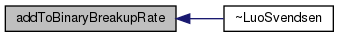The OpenFOAM FoundationLuoSvendsen Class Reference

Model of Luo and Svendsen (1996). The breakup rate is calculated by. More...

Inheritance diagram for LuoSvendsen: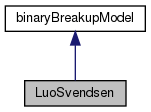[legend]
Collaboration diagram for LuoSvendsen: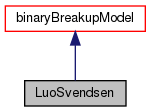[legend]

## Public Member Functions

TypeName ("LuoSvendsen")
Runtime type information. More...

LuoSvendsen (const populationBalanceModel &popBal, const dictionary &dict)

virtual ~LuoSvendsen ()
Destructor. More...

virtual void correct ()
Correct diameter independent expressions. More...

virtual void addToBinaryBreakupRate (volScalarField &binaryBreakupRate, const label i, const label j)Public Member Functions inherited from binaryBreakupModel
TypeName ("binaryBreakupModel")
Runtime type information. More...

declareRunTimeSelectionTable (autoPtr, binaryBreakupModel, dictionary,(const populationBalanceModel &popBal, const dictionary &dict),(popBal, dict))

binaryBreakupModel (const populationBalanceModel &popBal, const dictionary &dict)

autoPtr< binaryBreakupModelclone () const

virtual ~binaryBreakupModel ()
Destructor. More...Static Public Member Functions inherited from binaryBreakupModel
static autoPtr< binaryBreakupModelNew (const word &type, const populationBalanceModel &popBal, const dictionary &dict)Protected Attributes inherited from binaryBreakupModel
const populationBalanceModelpopBal_
Reference to the populationBalanceModel. More...

## Detailed Description

Model of Luo and Svendsen (1996). The breakup rate is calculated by.where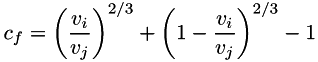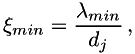and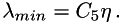The integral in the first expression is solved by means of incomplete Gamma functions as given by Bannari et al. (2008):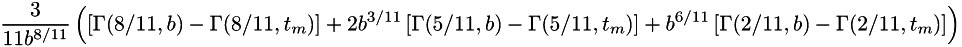where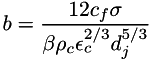and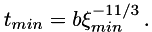Note that in the code, the upper incomplete gamma function is expressed as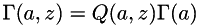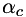= Void fraction of continuous phase [-]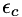= Turbulent dissipation rate of continuous phase [m^2/s^3]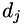= Diameter of mother bubble j [m^3]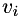= Volume of daughter bubble i [m^3]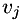= Volume of mother bubble j [m^3]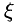= Integration variable [-]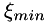= Lower bound of integral [-]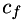= Increase coefficient of surface area [-]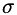= Surface tension [N/m]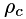= Density of continuous phase [kg/m^3]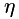= Kolmogorov length scale [m]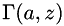= Upper incomplete gamma function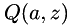= Regularized upper incomplete gamma function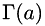= Gamma function

References:

        Luo, H., & Svendsen, H. F. (1996).
Theoretical model for drop and bubble breakup in turbulent dispersions.
AIChE Journal, 42(5), 1225-1233.
Eq. 27, p. 1229.
        Bannari, R., Kerdouss, F., Selma, B., Bannari, A., & Proulx, P. (2008).
Three-dimensional mathematical modeling of dispersed two-phase flow
using class method of population balance in bubble columns.
Computers & chemical engineering, 32(12), 3224-3237.
Eq. 49, p. 3230.
Usage
Property Description Required Default value
C4 Coefficient C4 no 0.923
beta Coefficient beta no 2.05
C5 Minimum eddy ratio no 11.4
Source files

Definition at line 228 of file LuoSvendsen.H.

## ◆ LuoSvendsen()

 LuoSvendsen ( const populationBalanceModel & popBal, const dictionary & dict )

## ◆ ~LuoSvendsen()

 virtual ~LuoSvendsen ( )
inlinevirtual

Destructor.

Definition at line 274 of file LuoSvendsen.H.

Here is the call graph for this function:## ◆ TypeName()

 TypeName ( "LuoSvendsen" )

Runtime type information.

## ◆ correct()

 virtual void correct ( )
virtual

Correct diameter independent expressions.

Reimplemented from binaryBreakupModel.

Referenced by LuoSvendsen::~LuoSvendsen().

Here is the caller graph for this function: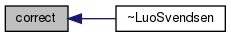virtual void addToBinaryBreakupRate ( volScalarField & binaryBreakupRate, const label i, const label j )
virtual